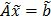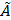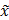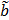Archive
Special IssuesVolume 5, Issue 2, June 2019, Page: 40-46
Algebraic Modification of Trapezoidal Fuzzy Numbers to Complete Fully Fuzzy Linear Equations System Using Gauss-Jacobi Method
Ahmad Syaiful Abidin, Department of Mathematics, University of Riau, Pekanbaru, Indonesia
Sri Gemawati, Department of Mathematics, University of Riau, Pekanbaru, Indonesia
Received: Jul. 20, 2019;       Accepted: Aug. 19, 2019;       Published: Sep. 2, 2019
Abstract
This paper will discuss algebraic modification of trapezoidal fuzzy numbers with a general form of fully fuzzy numbers iswithis n × n fuzzy matrix,fuzzy vector, andunknown fuzzy vector. The concept used in this paper is define positive or negative fuzzy numbers determined by the area on the left side of the x-axis and the right side of the x-axis. Furthermore, the concept will be applied to the multiplication of two fully fuzzy trapezoidal numbers to produce a new algebra that can be applied to a system of linear equations. At the end, an example of multiplication two fully fuzzy trapezoidal numbers using the Gauss-Jacobi method will be given. As a result compatible number will be obtained.
Keywords
Fully Fuzzy Number, Trapezoidal, New Algebra, Gauss-Jacobi Method
Ahmad Syaiful Abidin, Mashadi Mashadi, Sri Gemawati, Algebraic Modification of Trapezoidal Fuzzy Numbers to Complete Fully Fuzzy Linear Equations System Using Gauss-Jacobi Method, International Journal of Management and Fuzzy Systems. Vol. 5, No. 2, 2019, pp. 40-46. doi: 10.11648/j.ijmfs.20190502.12
Reference

T. Allahviranloo, S. Salahshour, M. Homayoun-Nejad dan D. Beleanu, General solution of fully fuzzy systems, Abstract and Applied Analysis, 2013, 2013, 1-9.

A. jafarian, New decomposition method for solving dual fully fuzzy linear system, Imt. Journal Fuzzy Computation and Modelling, 2, 2016, 76-85.

M. Deghan dan B. Hashemi, Iterative solution of fuzzy linear system, Applied Mathematics and Computation, 175, 2006, 645-674.

S. Gemawati, Nasﬁanti, Mashadi dan A Hadi, A new for dual fully fuzzy linear system with trapezoidal fuzzy number by QR decomposition, Journal of Physics, 1116, 2018, 1-5.

G. Malkawi, N. Ahmad dan H. Ibrahim, Solving fully fuzzy linear sys tem with the necessary an suficient to have a positive solution, Applied Mathematics and Information Sciences, 8, 2014, 1003-1019.

A. Hadi, Mashadi dan S. Gemawati, On fuzzy n-inner product spaces, Journal of Physics, 020010, 2017, 1-6.

S. I. Marni, Mashadi, S. Gemawati, Solving dual fully fuzzy linear system by use factorizations of the coeficient matrix for trapezoidal fuzzy number, Bulletin of Mathematics, 2, 2018, 145-156.

N. J. Karthik dan E. Chandrasekaran, Solving fully fuzzy linear system with trapezoidal fuzzy number matrices by partitioning, International Jo- urnal of Computer Applications, 64, 2013, 35-38.

A. Kumar, Neetu, dan A. Bansal, A new method to solve fully fuzzy linear system with trapezoidal fuzzy number, Canadian Journal on Science and Engineering Mathematics, 1, 2010, 45-56.

A. Kumar, J. Kaur dan P singh, A new method for solving fuzzy linier programs with trapezoidal fuzzy numbers, Journal of Fuzzy, 2011, 2011, 1-12.

A. Kumar and J. Kaur, Comentary on “Calculating fuzzy inverse matrix using fuzzy linear equation system”, Applied Soft Computing, 58, 2017, 324-327.

L. A. Zadeh, Fuzzy Sets, Information and Control, 8, 1965, 338-353.

L. Abdullah dan N. A. Rahman, Jacobi-based methods in solving fuzzy linier system, International Journal of Mathematical and Computational Science, 7, 2013, 402-408.

Mashadi, A new method for dual fully fuzzy linier system by use LU factorizations of the coeficient matrix, Jurnal Matematika dan Sains, 15, 2010, 101-106.

S. Moloudzadeh, P. Darabi dan H. Khandani, The pseudo invers matrices to solve general fully fuzzy linear system, Journal of Soft Computing and Applications, 2013, 2013, 1-11.

S. H. Nasheri dan M. Gholami, Linear system of equation with trapezoidal fuzzy numbers, The Journal of Mathematics and Computer Science, 3, 2011, 71-79.

S. Das dan S. Chakraverty, Numerical solution of fuzzy system of linear equation, Applications and Applied Mathematics, 7, 2012, 334-356.